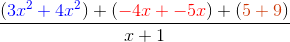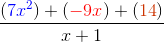## Example Questions

### Example Question #1 : How To Add Rational Expressions With A Common Denominator

Compute the following: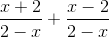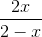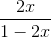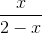Explanation:

Notice that the denominator are the same for both terms. Since they are both the same, the fractions can be added. The denominator will not change in this problem.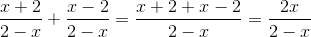### Example Question #1 : How To Add Rational Expressions With A Common Denominator

Simplify the following rational expression: (9x - 2)/(x2) MINUS (6x - 8)/(x2)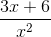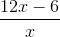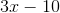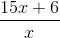Explanation:

Since both expressions have a common denominator, x2, we can just recopy the denominator and focus on the numerators. We get (9x - 2) - (6x - 8). We must distribute the negative sign over the 6x - 8 expression which gives us 9x - 2 - 6x + 8 ( -2 minus a -8 gives a +6 since a negative and negative make a positive). The numerator is therefore 3x + 6.

### Example Question #11 : Expressions

Simplify the following rational expression: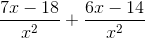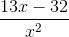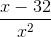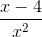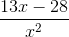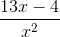Explanation:

Since both fractions in the expression have a common denominator of, we can combine like terms into a single numerator over the denominator: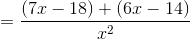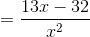### Example Question #4 : How To Add Rational Expressions With A Common Denominator

Simplify the following expression: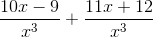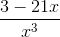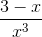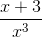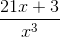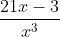Explanation:

Since both terms in the expression have the common denominator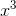, combine the fractions and simplify the numerators: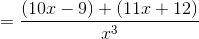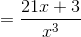### Example Question #3 : How To Add Rational Expressions With A Common Denominator

Simplify the following rational expression: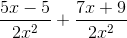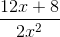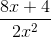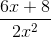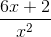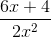Explanation:

Since both rational terms in the expression have the common denominator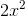, combine the numerators and simplify like terms: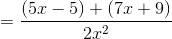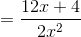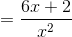### Example Question #2 : How To Add Rational Expressions With A Common Denominator

Combine the following rational expressions: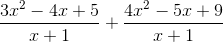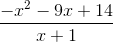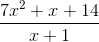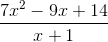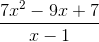Explanation:

When working with complex fractions, it is important not to let them intimidate you. They follow the same rules as regular fractions!In this case, our problem is made easier by the fact that we already have a common denominator. Nothing fancy is required to start. Simply add the numerators: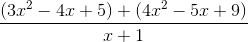For our next step, we need to combine like terms. This is easier to see if we group them together.